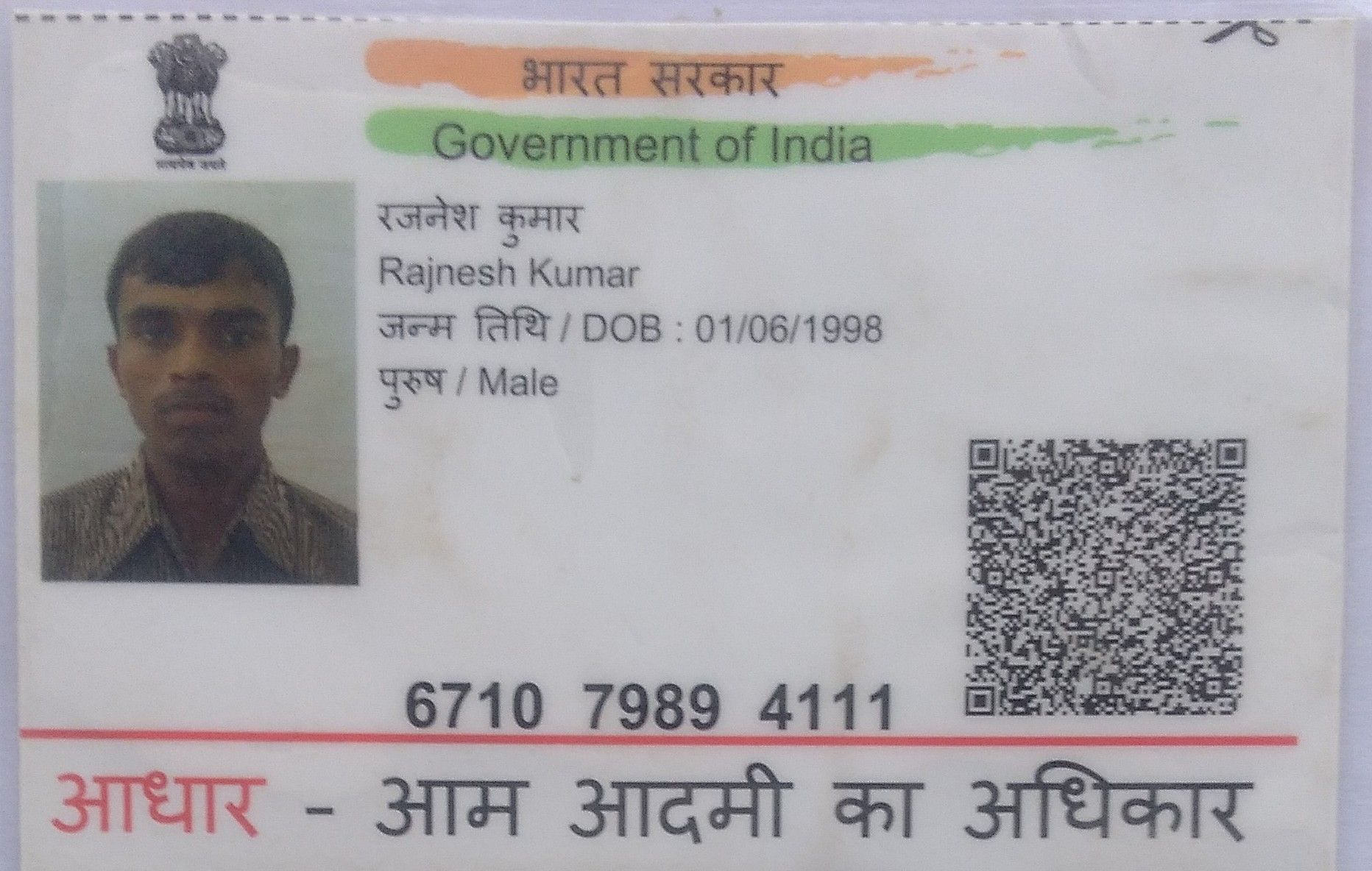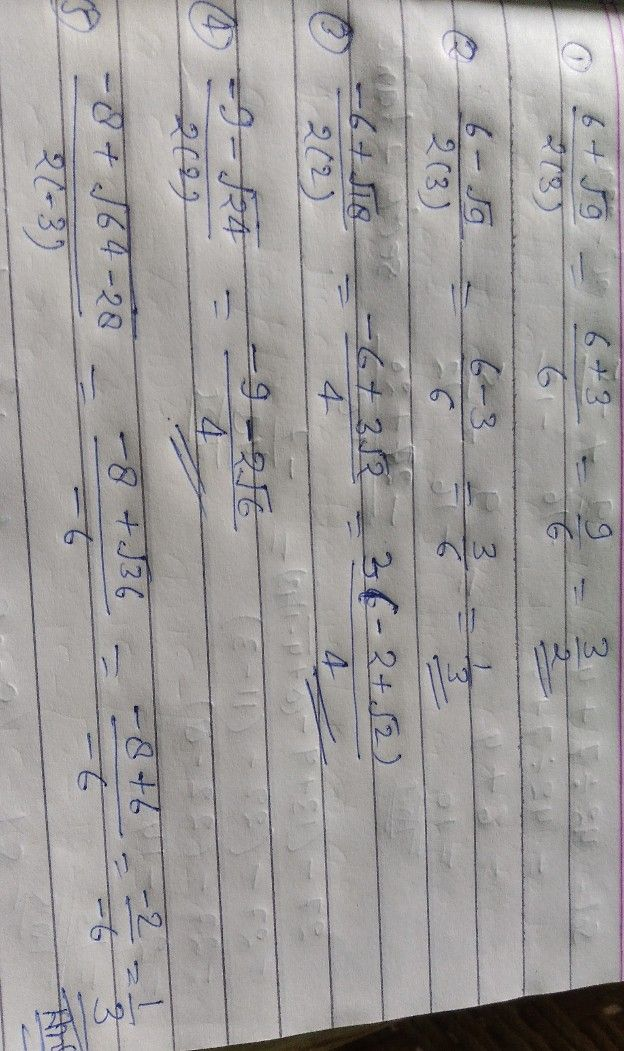Symbol
ProblemSimplify the following expressions. Answer the following questions that follow. $1$ $\dfrac {6+\sqrt{9} } {2\left(3\right)}$ $\dfrac {6-\sqrt{9} } {2\left(3\right)}$ $2$ $3$ $\dfrac {-6+\sqrt{18} } {2\left(2\right)}$ $4$ $\dfrac {-9-\sqrt{24} } {2\left(2\right)}$ hotuloe $5$ $\dfrac {-8+\sqrt{64-28} } {2\left(-3\right)}$
7th-9th grade
Other
Search count: 117
SolutionQanda teacher - rajneshStudent
thank youQanda teacher - rajnesh
ok